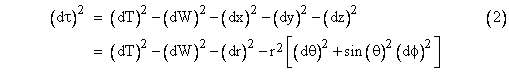# 7. Cosmology 1 Is the Universe Closed?

 Page 8/12 Date 26.04.2018 Size 0.57 Mb. #46938

## 7.6  Cosmological Coherence

Our main “difference in creed” is that you have a specific belief and I am a skeptic.

Willem de Sitter, 1917

Almost immediately after Einstein arrived at the final field equations of general relativity, the very foundation of his belief in those equations was shaken, first by appearance of Schwarzschild’s exact solution of the one-body problem. This was disturbing to Einstein because at the time he held the Machian belief that inertia must be attributable to the effects of distant matter, so he thought the only rigorous global solutions of the field equations would require some suitable distribution of distant matter. Schwarzschild’s solution represents a well-defined spacetime extending to infinity, with ordinary inertial behavior for infinitesimal test particles, even though the only significant matter in this universe is the single central gravitating body. That body influences the spacetime in its vicinity, but the metric throughout spacetime is primarily determined by the spherical symmetry, leading to asymptotically flat spacetime at great distances from the central body. This seems rather difficult to reconcile with “Mach’s Principle”, but there was worse to come, and it was Einstein himself who opened the door.

In an effort to conceive of a static cosmology with uniformly distributed matter he found it necessary to introduce another term to the field equations, with a coefficient called the cosmological constant. (See Section 5.8.) Shortly thereafter, Einstein received a letter from the astronomer Willem de Sitter, who pointed out a global solution of the modified field equations (i.e., with non-zero cosmological constant) that is entirely free of matter, and yet that possesses non-trivial metrical structure. This thoroughly un-Machian universe was a fore-runner of Gödel’s subsequent cosmological models containing closed-timelike curves. After a lively and interesting correspondence about the shape of the universe, carried on between a Dutch astronomer and a German physicist at the height of the first world war, de Sitter published a paper on his solution, and Einstein published a rebuttal, claiming (incorrectly) that “the De Sitter system does not look at all like a world free of matter, but rather like a world whose matter is concentrated entirely on the [boundary]”. The discussion was joined by several other prominent scientists, including Weyl, Klein, and Eddington, who all tried to clarify the distinction between singularities of the coordinates and actual singularities of the manifold/field. Ultimately all agreed that de Sitter was right, and his solution does indeed represent a matter-free universe consistent with the modified field equations.

We’ve seen that the Schwarzschild metric represents the unique spherically symmetrical solution of the original field equations of general relativity - assuming the cosmological constant, denoted by  in Section 5.8, is zero.  If we allow a non-zero value of , the Schwarzschild solution generalizes to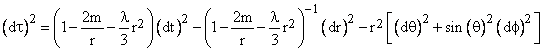To avoid upsetting the empirical successes of general relativity, such as the agreement with Mercury’s excess precession, the value of  must be extremely small, certainly less than 10-40 m-2, but not necessarily zero. If  is precisely zero, then the Schwarzschild metric goes over to the Minkowski metric when the gravitating mass m equals zero, but if  is not precisely zero the Schwarzschild metric with zero mass is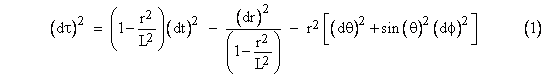where L is a characteristic length related to the cosmological constant by L2 = 3/. This is one way of writing the metric of de Sitter spacetime. Just as Minkowski spacetime is a solution of the original vacuum field equation R = 0, so the de Sitter metric is a solution of the modified field equations R = g. Since there is no central mass in this case, it may seem un-relativistic to use polar coordinates centered on one particular point, but it can be shown that – just as with the Minkowski metric in polar coordinates – the metric takes the same form when centered on any point.

The metric (1) can be written in a slightly different form in terms of the radial coordinate  defined by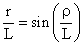Noting that dr/L = cos(/L)d, the de Sitter metric is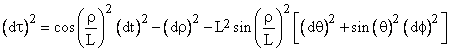Interestingly, with a suitable change of coordinates, this is actually the metric of the surface of a four-dimensional pseudo-sphere in five-dimensional Minkowski space. Returning to equation (1), let x,y,z denote the usual three orthogonal spatial coordinates such that x2 + y2 + z2 = r2, and suppose there is another orthogonal spatial coordinate W and a time coordinate T defined by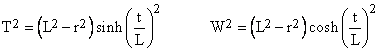For any values of x,y,z,t we have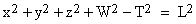so this locus of events comprises the surface of a hyperboloid, i.e., a pseudo-sphere of “radius” L. In other words, the spatial universe for any given time T is the three-dimensional surface of the four-dimensional sphere of squared radius L2 + T2. Hence the space shrinks to a minimum radius L at time T = 0 and then expands again as T increases, as illustrated below (showing only two of the spatial dimensions).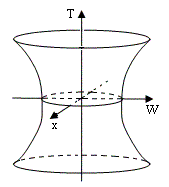Assuming the five-dimensional spacetime x,y,z,W,T has the Minkowski metric